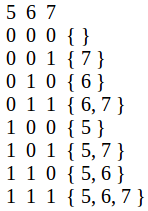Skip to content
Related Articles
Find sum of sum of all sub-sequences
• Difficulty Level : Easy
• Last Updated : 09 Jun, 2021

Given an array of n integers. The task is to find the sum of each sub-sequence of the array.
Examples :

```Input : arr[] = { 6, 8, 5 }
Output : 76
All subsequence sum are:
{ 6 }, sum = 6
{ 8 }, sum = 8
{ 5 }, sum = 5
{ 6, 8 }, sum = 14
{ 6, 5 }, sum = 11
{ 8, 5 }, sum = 13
{ 6, 8, 5 }, sum = 19
Total sum = 76.

Input  : arr[] = {1, 2}
Output : 6```

Method 1 (brute force):
Generate all the sub-sequence and find the sum of each sub-sequence.
Method 2 (efficient approach):
For an array of size n, we have 2^n sub-sequences (including empty) in total. Observe, in total 2n sub-sequences, each element occurs 2n-1 times.
For example, arr[] = { 5, 6, 7 }So, the sum of all sub-sequence will be (sum of all the elements) * 2n-1.
Below is the implementation of this approach:

## C++

 `// C++ program to find sum of all sub-sequences``// of an array.``#include``using` `namespace` `std;` `// Return sum of sum of all sub-sequence.``int` `sum(``int` `arr[], ``int` `n)``{``  ``int` `ans = 0;` `  ``// Finding sum of the array.``  ``for` `(``int` `i = 0; i < n; i++)``    ``ans += arr[i];` `  ``return` `ans * ``pow``(2, n - 1);``}` `// Driver Code``int` `main()``{``  ``int` `arr[] = { 6, 7, 8 };``  ``int` `n = ``sizeof``(arr)/``sizeof``(arr);` `  ``cout << sum(arr, n) << endl;` `  ``return` `0;``}`

## Java

 `// Java program to find sum of``// all sub-sequences of an array.``import` `java.io.*;``import` `java.math.*;` `class` `GFG {``    ` `    ``// Return sum of sum of all sub-sequence.``    ``static` `int` `sum(``int` `arr[], ``int` `n)``    ``{``    ``int` `ans = ``0``;``    ` `    ``// Finding sum of the array.``    ``for` `(``int` `i = ``0``; i < n; i++)``        ``ans += arr[i];``    ` `    ``return` `ans * (``int``)(Math.pow(``2``, n - ``1``));``    ``}``    ` `    ``// Driver Code``    ``public` `static` `void` `main(String args[])``    ``{``    ``int` `arr[]= { ``6``, ``7``, ``8` `};``    ``int` `n = arr.length;``    ` `    ``System.out.println(sum(arr, n));``    ``}``}``    ` `// This code is contributed by Nikita Tiwari.`

## Python3

 `# Python 3 program to find sum of``# all sub-sequences of an array.`  `# Return sum of sum of all sub-sequence.``def` `sm(arr , n) :``    ``ans ``=` `0` `    ``# Finding sum of the array.``    ``for` `i ``in` `range``(``0``, n) :``        ``ans ``=` `ans ``+` `arr[i]``    ` `    ``return` `ans ``*` `pow``(``2``, n ``-` `1``)``    ` `    ` `# Driver Code``arr ``=` `[ ``6``, ``7``, ``8` `]``n``=``len``(arr)` `print``(sm(arr, n))`  `# This code is contributed by Nikita Tiwari.`

## C#

 `// C# program to find sum of``// all sub-sequences of an array.``using` `System;` `class` `GFG``{``    ` `    ``// Return sum of sum of all sub-sequence.``    ``static` `int` `sum(``int` `[]arr, ``int` `n)``    ``{``    ``int` `ans = 0;``    ` `    ``// Finding sum of the array.``    ``for` `(``int` `i = 0; i < n; i++)``        ``ans += arr[i];``    ` `    ``return` `ans * (``int``)(Math.Pow(2, n - 1));``    ``}``    ` `    ``// Driver Code``    ``public` `static` `void` `Main()``    ``{``    ``int` `[]arr= { 6, 7, 8 };``    ``int` `n = arr.Length;``    ` `    ``Console.Write(sum(arr, n));``    ``}``}``    ` `// This code is contributed by nitin mittal`

## PHP

 ``

## Javascript

 ``

Output:

`84`

This article is contributed by Anuj Chauhan. If you like GeeksforGeeks and would like to contribute, you can also write an article using write.geeksforgeeks.org or mail your article to review-team@geeksforgeeks.org. See your article appearing on the GeeksforGeeks main page and help other Geeks.
Please write comments if you find anything incorrect, or you want to share more information about the topic discussed above.

Attention reader! Don’t stop learning now. Get hold of all the important DSA concepts with the DSA Self Paced Course at a student-friendly price and become industry ready.  To complete your preparation from learning a language to DS Algo and many more,  please refer Complete Interview Preparation Course.

In case you wish to attend live classes with industry experts, please refer Geeks Classes Live

My Personal Notes arrow_drop_up